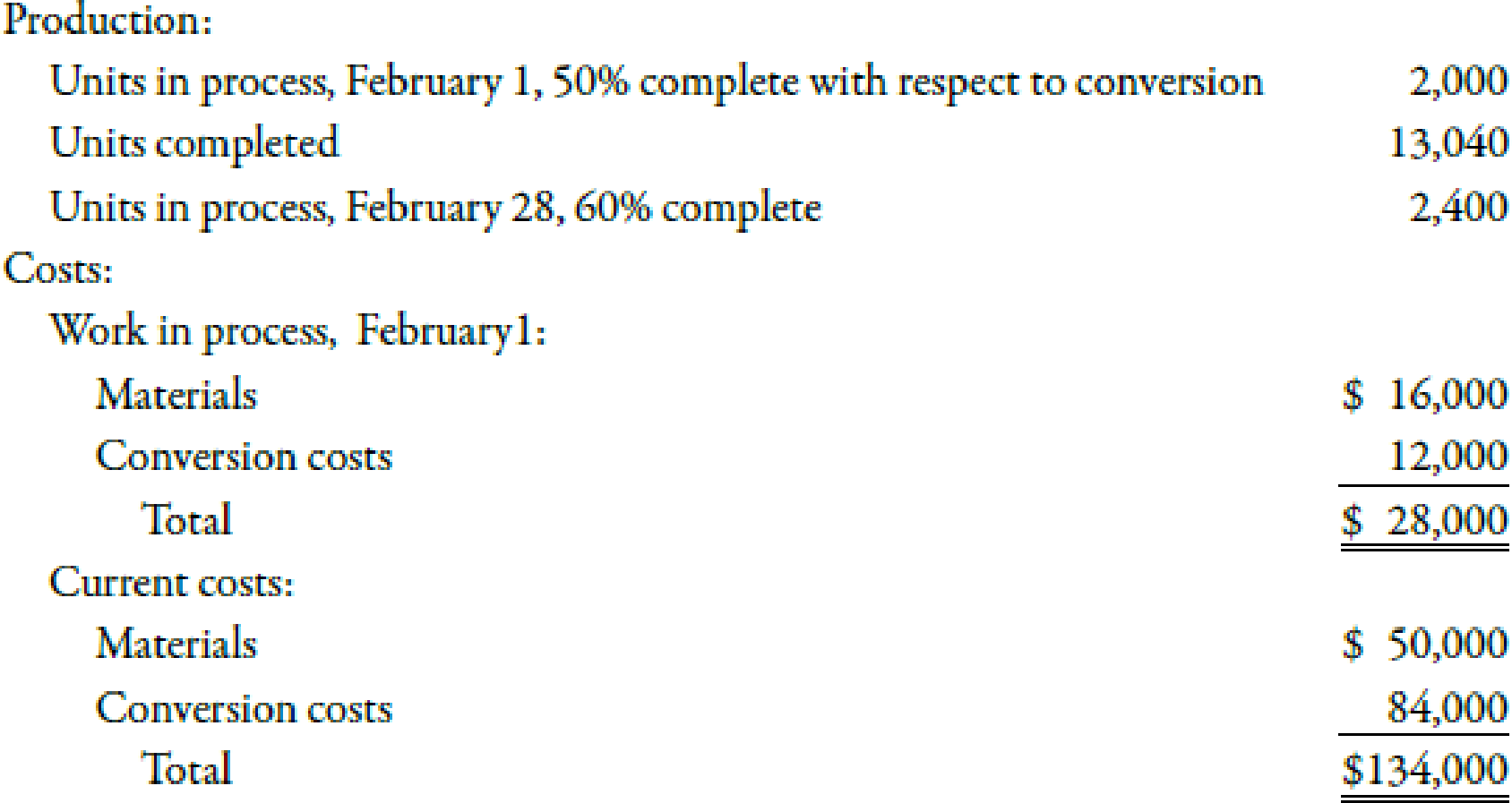Chapter 6, Problem 37BEB### Managerial Accounting: The Corners...

7th Edition
Maryanne M. Mowen + 2 others
ISBN: 9781337115773

#### Solutions

Chapter
Section### Managerial Accounting: The Corners...

7th Edition
Maryanne M. Mowen + 2 others
ISBN: 9781337115773
Textbook Problem
1 views

# Nonuniform Inputs, Weighted AverageMing Inc. had the following production and cost information for its blending department during February (with materials added at the beginning of the process):Ming uses the weighted average method.Required: 1. Prepare an equivalent units schedule. 2. Calculate the unit cost. (Note: Round answers to two decimal places.) 3. Calculate the cost of units transferred out and the cost of EWIP.

1.

To determine

Compute the equivalent units of output with the help of equivalent schedule.

Explanation

Equivalent Units of Production:

The total units of finished goods along with those goods that are not fully complete are known as equivalent units of production. In other words, equivalent units of production measures both completed and nearly completed units.

Prepare the table to show the calculation of equivalent units as given below:

<
 Particulars Materials Conversion Units completed 13,040 13,040 Add: Units in Ending Work in Process × Fraction Complete:

2.

To determine

Compute the amount of unit cost.

3.

To determine

Identify the cost of units transferred out and the cost of EWIP.

### Still sussing out bartleby?

Check out a sample textbook solution.

See a sample solution

#### The Solution to Your Study Problems

Bartleby provides explanations to thousands of textbook problems written by our experts, many with advanced degrees!

Get Started